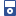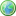Accessibility options:

# Vectors resources

Show me all resources applicable to

### iPOD Video (14)Introduction to vectors - 1
A vector is a quantity that has both a magnitude (or size) and a direction. Both of these properties must be given in order to specify a vector completely. In this unit we describe how to write down vectors, how to add and subtract them, and how to use them in geometry. This resource is released under a Creative Commons license Attribution-Non-Commercial-No Derivative Works and the copyright is held by Skillbank Solutions Ltd.Introduction to vectors - 2
A vector is a quantity that has both a magnitude (or size) and a direction. Both of these properties must be given in order to specify a vector completely. In this unit we describe how to write down vectors, how to add and subtract them, and how to use them in geometry. This resource is released under a Creative Commons license Attribution-Non-Commercial-No Derivative Works and the copyright is held by Skillbank Solutions Ltd.Introduction to vectors - 3
A vector is a quantity that has both a magnitude (or size) and a direction. Both of these properties must be given in order to specify a vector completely. In this unit we describe how to write down vectors, how to add and subtract them, and how to use them in geometry. This resource is released under a Creative Commons license Attribution-Non-Commercial-No Derivative Works and the copyright is held by Skillbank Solutions Ltd.Introduction to vectors - 4
A vector is a quantity that has both a magnitude (or size) and a direction. Both of these properties must be given in order to specify a vector completely. In this unit we describe how to write down vectors, how to add and subtract them, and how to use them in geometry. This resource is released under a Creative Commons license Attribution-Non-Commercial-No Derivative Works and the copyright is held by Skillbank Solutions Ltd.Vectors - Scalar Product 1
One of the ways in which two vectors can be combined is known as the scalar product. When we calculate the scalar product of two vectors the result, as the name suggests is a scalar, rather than a vector. This resource is released under a Creative Commons license Attribution-Non-Commercial-No Derivative Works and the copyright is held by Skillbank Solutions Ltd.Vectors - Scalar Product 2
One of the ways in which two vectors can be combined is known as the scalar product. When we calculate the scalar product of two vectors the result, as the name suggests is a scalar, rather than a vector. This resource is released under a Creative Commons license Attribution-Non-Commercial-No Derivative Works and the copyright is held by Skillbank Solutions Ltd.Vectors - Scalar Product 3
One of the ways in which two vectors can be combined is known as the scalar product. When we calculate the scalar product of two vectors the result, as the name suggests is a scalar, rather than a vector. This resource is released under a Creative Commons license Attribution-Non-Commercial-No Derivative Works and the copyright is held by Skillbank Solutions Ltd.Vectors - Scalar Product 4
One of the ways in which two vectors can be combined is known as the scalar product. When we calculate the scalar product of two vectors the result, as the name suggests is a scalar, rather than a vector. This resource is released under a Creative Commons license Attribution-Non-Commercial-No Derivative Works and the copyright is held by Skillbank Solutions Ltd.Vectors - Scalar Product 5
One of the ways in which two vectors can be combined is known as the scalar product. When we calculate the scalar product of two vectors the result, as the name suggests is a scalar, rather than a vector. This resource is released under a Creative Commons license Attribution-Non-Commercial-No Derivative Works and the copyright is held by Skillbank Solutions Ltd.Vectors - The Vector Product 1
One of the ways in which two vectors can be combined is known as the vector product. When we calculate the vector product of two vectors the result, as the name suggests, is a vector. This resource is released under a Creative Commons license Attribution-Non-Commercial-No Derivative Works and the copyright is held by Skillbank Solutions Ltd.Vectors - The Vector Product 2
One of the ways in which two vectors can be combined is known as the vector product. When we calculate the vector product of two vectors the result, as the name suggests, is a vector. This resource is released under a Creative Commons license Attribution-Non-Commercial-No Derivative Works and the copyright is held by Skillbank Solutions Ltd.Vectors - The Vector Product 3
One of the ways in which two vectors can be combined is known as the vector product. When we calculate the vector product of two vectors the result, as the name suggests, is a vector. This resource is released under a Creative Commons license Attribution-Non-Commercial-No Derivative Works and the copyright is held by Skillbank Solutions Ltd.Vectors - The Vector Product 4
One of the ways in which two vectors can be combined is known as the vector product. When we calculate the vector product of two vectors the result, as the name suggests, is a vector. This resource is released under a Creative Commons license Attribution-Non-Commercial-No Derivative Works and the copyright is held by Skillbank Solutions Ltd.Vectors - The Vector Product 5
One of the ways in which two vectors can be combined is known as the vector product. When we calculate the vector product of two vectors the result, as the name suggests, is a vector. This resource is released under a Creative Commons license Attribution-Non-Commercial-No Derivative Works and the copyright is held by Skillbank Solutions Ltd.

### Quick Reference (3)The scalar product
This leaflet defines the scalar product of two vectors and gives some examples. It shows how the scalar product can be used to find the angle between two vectors. (Engineering Maths First Aid Kit 6.2)The vector product
This leaflet defines the vector product of two vectors and gives some examples. It shows how the vector product can be evaluated using determinants. (Engineering Maths First Aid Kit 6.3)Vectors
This leaflet explains notations in common use for describing vectors, and shows how to calculate the modulus of vectors given in Cartesian form. (Engineering Maths First Aid Kit 6.1)

### Teach Yourself (4)Cartesian components of vectors
Any vector may be expressed in Cartesian components, by using unit vectors in the directions of the coordinate axes. In this unit we describe these unit vectors in two dimensions and in three dimensions, and show how they can be used in calculations.Introduction to vectors
A vector is a quantity that has both a magnitude (or size) and a direction. Both of these properties must be given in order to specify a vector completely. In this unit we describe how to write down vectors, how to add and subtract them, and how to use them in geometry.The scalar product
One of the ways in which two vectors can be combined is known as the scalar product. When we calculate the scalar product of two vectors the result, as the name suggests is a scalar, rather than a vector.The vector product
One of the ways in which two vectors can be combined is known as the vector product. When we calculate the vector product of two vectors the result, as the name suggests, is a vector.

### Test Yourself (3)Dot and cross product - Numbas
5 questions on vectors. Scalar product, angle between vectors, cross product, when are vectors perpendicular, combinations of vectors defined or not. Numbas resources have been made available under a Creative Commons licence by Bill Foster and Christian Perfect, School of Mathematics & Statistics at Newcastle University.Maths EG
Computer-aided assessment of maths, stats and numeracy from GCSE to undergraduate level 2. These resources have been made available under a Creative Common licence by Martin Greenhow and Abdulrahman Kamavi, Brunel University.Vector Test 01 (DEWIS)
Five questions on vectors, testing addition, subtraction, scalar multiplication, magnitude, scalar product, vector product and finding the angle between two vectors. DEWIS resources have been made available under a Creative Commons licence by Rhys Gwynllyw & Karen Henderson, University of the West of England, Bristol.

### Third Party Resources (1)Mathematics Support Materials from the University of Plymouth
Support material from the University of Plymouth:
The output from this project is a library of portable, interactive, web based support packages to help students learn various mathematical ideas and techniques and to support classroom teaching.
There are support materials on ALGEBRA, GRAPHS, CALCULUS, and much more.
This material is offered through the mathcentre site courtesy of Dr Martin Lavelle and Dr Robin Horan from the University of Plymouth.

### Video (4)Cartesian components of vectors
Any vector may be expressed in Cartesian components, by using unit vectors in the directions of the coordinate axes. In this unit we describe these unit vectors in two dimensions and in three dimensions, and show how they can be used in calculations. (Mathtutor Video Tutorial). This resource is released under a Creative Commons license Attribution-Non-Commercial-No Derivative Works and the copyright is held by Skillbank Solutions Ltd.Introduction to vectors
A vector is a quantity that has both a magnitude (or size) and a direction. Both of these properties must be given in order to specify a vector completely. In this unit we describe how to write down vectors, how to add and subtract them, and how to use them in geometry. (Mathtutor Video Tutorial) This resource is released under a Creative Commons license Attribution-Non-Commercial-No Derivative Works and the copyright is held by Skillbank Solutions Ltd.The scalar product
One of the ways in which two vectors can be combined is known as the scalar product. When we calculate the scalar product of two vectors the result, as the name suggests is a scalar, rather than a vector. (Mathtutor Video Tutorial) This resource is released under a Creative Commons license Attribution-Non-Commercial-No Derivative Works and the copyright is held by Skillbank Solutions Ltd.The vector product
One of the ways in which two vectors can be combined is known as the vector product. When we calculate the vector product of two vectors the result, as the name suggests, is a vector. (Mathtutor Video Tutorial) This resource is released under a Creative Commons license Attribution-Non-Commercial-No Derivative Works and the copyright is held by Skillbank Solutions Ltd.

Website design by Pink Mayhem, Leicester